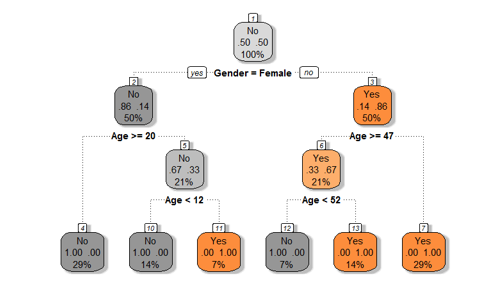• No products in the cart.

# 203.3.9 The Problem of Over fitting the Decision Tree

### LAB: The Problem of Over fitting

In previous section, we studied about Validating the Tree

• Import both test and training data
• Build a decision tree model on training data
• Find the accuracy on training data
• Find the predictions for test data
• What is the model prediction accuracy on test data?

### Solution

• Import both test and training data
``````Train <- read.csv("C:\Amrita\Datavedi\Buyers Profiles\Train_data.csv")
• Build a decision tree model on training data
``````buyers_model<-rpart(Bought ~ Age + Gender, method="class", data=Train,control=rpart.control(minsplit=2))
``````## n= 14
##
## node), split, n, loss, yval, (yprob)
##       * denotes terminal node
##
##  1) root 14 7 No (0.5000000 0.5000000)
##    2) Gender=Female 7 1 No (0.8571429 0.1428571)
##      4) Age>=20 4 0 No (1.0000000 0.0000000) *
##      5) Age< 20 3 1 No (0.6666667 0.3333333)
##       10) Age< 11.5 2 0 No (1.0000000 0.0000000) *
##       11) Age>=11.5 1 0 Yes (0.0000000 1.0000000) *
##    3) Gender=Male 7 1 Yes (0.1428571 0.8571429)
##      6) Age>=47 3 1 Yes (0.3333333 0.6666667)
##       12) Age< 52 1 0 No (1.0000000 0.0000000) *
##       13) Age>=52 2 0 Yes (0.0000000 1.0000000) *
##      7) Age< 47 4 0 Yes (0.0000000 1.0000000) *``````
• Find the accuracy on training data
``````predicted_values<-predict(buyers_model,type="class")
actual_values<-Train\$Bought

conf_matrix<-table(predicted_values,actual_values)
accuracy<-(conf_matrix[1,1]+conf_matrix[2,2])/(sum(conf_matrix))
accuracy``````
``##  1``
• Find the predictions for test data
``````predicted_values<-predict(buyers_model,type="class",newdata=Test)
predicted_values``````
``````##   1   2   3   4   5   6
##  No  No  No Yes  No Yes
## Levels: No Yes``````

What is the model prediction accuracy on test data?

``````actual_values<-Test\$Bought

conf_matrix<-table(predicted_values,actual_values)
accuracy<-(conf_matrix[1,1]+conf_matrix[2,2])/(sum(conf_matrix))
accuracy``````
``##  0.3333333``

### The Final Tree with Rules

1. Gender=Female & Age>=20 No *
1. Gender=Female & Age< 20 & Age< 11.5 No *
1. Gender=Female & Age< 20 & Age>=11.5 Yes *
1. Gender=Male & Age>=47 & Age< 52 No *
1. Gender=Male & Age>=47 & Age>=52 Yes *
1. Gender=Male & Age< 47 Yes *### The Problem of Overfitting

• If we further grow the tree we might even see each row of the input data table as the final rules
• The model will be really good on the training data but it will fail to validate on the test data
• Growing the tree beyond a certain level of complexity leads to overfitting
• A really big tree is very likely to suffer from overfitting.

The next post is about Pruning a Decision tree in R.

20th June 2017

### 0 responses on "203.3.9 The Problem of Over fitting the Decision Tree"

#### Statinfer

Statinfer derived from Statistical inference is a company that focuses on the data science training and R&D.We offer training on Machine Learning, Deep Learning and Artificial Intelligence using tools like R, Python and TensorFlow